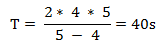New Student User - Use Code HELLO

# Problems based on Trains: Rules and Tricks

Some concepts of the time speed, distance and time are applicable to these problems also.
Relative Speed: - The speed of an object with respect to other objects which may be stationary or moving in either same or opposite direction.

## Rules to solve such problems

### Rule 1:

Relative Speed (S):
I. Relative Speed of a stationary object and moving object = Speed of the moving object.
II. Relative Speed of two objects moving in opposite direction = Sum of their speeds.
III. Relative Speed of two objects moving in same direction = Difference of their speeds.

### Rule 3:

Distance Covered (d):
I.
Distance covered by train in passing a pole or a stationary man or a signal post or any other object of negligible length = Length of the train.
II. Distance covered by train in passing a stationary object (bridge, platform etc) having same length = Sum of lengths of the train and that particular stationary object.

### Rule 4:

Time taken (t):
I.
Two trains of length and y are moving in opposite direction with speed u and v respectively, then time taken by trains to cross each other =
II. Two trains of length and y are moving in same direction with speed u and v respectively, then time taken by the faster train to cross the slower train=

## Formulas Including Short Tricks:

### Trick 1:

Calculation of time taken(t2) by train of length (L) passes a platform of length (y), if the same train passes another platform of length (x) in time(tt) –Example: - A train of length 250m passes a platform of length 350m in 50s then what time will this train take to pass the platform of 230m length?

### Trick 2:

Calculation of distance (d) from starting point where two trains meet, if two trains leave starting point at time t1& t2 and travel with the speeds of a & b respectively -Example: - Two trains leave Mumbai for Delhi at 11:00AM and 11:30AM respectively and travel at 120km/hr & 140km/hr respectively. How many kilometres from Mumbai, will the two trains meet?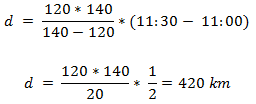### Trick 3:

Calculation of length of train (L), if a train overtakes two persons who are walking with the speeds of a & b respectively, in the same direction and train passes the two persons completely in time t1 & t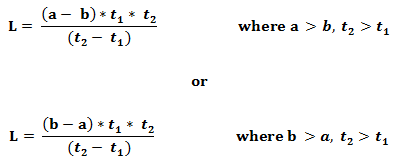Example: -A train overtakes two persons who are walking at the rate of 4m/s & 8m/s in the same direction and pass them completely in 18s & 20s respectively. Find the length of the train?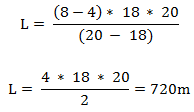### Trick 4:

Calculation of the speeds of faster and slower train–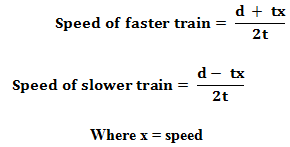Where x = speed
Example: - The distance between two stations P & Q is 140km. A train starts from P to Q and another starts from Q to P at the same time & they meet after 6h. The train travelling from P to Q is slower by 5km/hr compared to other trains from Q to P then find the speed of the slower train?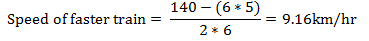### Trick 5:

Calculation the distance and speed of the train if speed of the train is reduced by ‘a’ km/hr –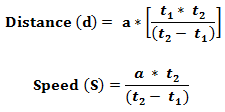Example:  -A train covers distance between two stations A & B in 3hr. if the speed of the train is reduced by 6km/hr then it travels the same distance in 4h. Calculate the distance between two stations & speed of the train?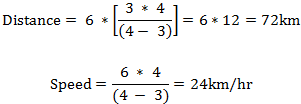### Trick 6:

Calculation of time of rest per hour–Example:  -Without stoppage, the speed of the train is 54km/hr and with stoppage, it is 45km/hr. For how many minutes, does the train stop per hour?### Trick 7:

Calculation of time taken by trains to cross each other, if two trains having equal length and different speeds take time t1 & t2  to cross a pole –
I. If trains are moving in opposite direction: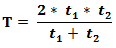II. If trains are moving in same direction:Example: -Two trains of equal lengths take 4s & 5s respectively to cross a pole. If these trains are moving in the same direction then how long will they to cross each other?